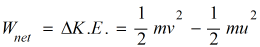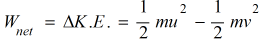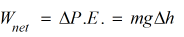Work and Energy homework Help at TutorEye

# Best Homework Help For Work and Energy

## Work and Energy:

The concept of work is described, when a force acting on an object causes displacement in any direction. The main components for the work to be defined are force and displacement.It is governed by Newton's law of motion, which serves as a model for analyzing motion of any body in terms of work done. Whereas, Energy is the ability to do work. In classical Mechanics, It is quantified by the potential and kinetic energy associated with the body at rest or in motion.

## Work and Energy Sample Questions:

Question 1: A body of mass 2 kg falls freely from a height of 5m. Find the amount of total work done during the process.

a) 1 N        b) 0 N

c) 2 N        d) 3 N

Explanation: Work done in a free fall

Question 2: Which of the following is a correct representation of the work energy theorem?

a)b)c)d) None of the above

Answer:  a)Explanation: The net work done by the net forces on a particle is equal to the change in kinetic energy

Question 3: For a perfectly inelastic collision, the total kinetic energy before the collision is _________ than the total kinetic energy after the collision.

a) less              b) equal

c) greater           d) cannot be defined

Explanation: In a perfectly inelastic collision

Question 4:  For a perfectly elastic bodies, coefficient of restitution is

a) 0            b) 0.5

c) 0.1          d) 1

Explanation: Given,

Question 5: A  body of mass 1 kg is travelling with a velocity of 10 m/s strikes an identical body B.What is the velocity of ball B after the impact, if the impact is perfectly elastic.

a) 10 m/s          b) 5 m/s

c) 2.5 m/s         d) 20 m/s

Explanation: Given,

Question 6: Virtual work concept can be applied to

a) Lifting bodies             b) Trusses

c) Beams                      d) All of the above

Answer: d) All of the above

Explanation:  The concept of virtual work states that in equilibrium the virtual work of the forces applied to a system is zero.

Question 7: During the inelastic impact of two bodies, which of the following statements is correct ?

a) Energy and momentum are conserved                           b) Energy is not conserved, momentum is conserved

c) Energy is conserved, momentum is not conserved         d) Neither momentum nor energy is conserved

Explanation: In a pure rolling motion, the relative velocity at point of contact is always zero

Question 8: A body of mass 50g moves with varying distance . The total work done by forces during the displacement x = 0 to x = 4

a) 1.5 J           b) 2 J

c) 2.5 J           d) 3 J

Explanation: Given,

Question 9: Work energy theorem is defined for

a) Internal force         b) Conservative force

c) External force        d) All of the above

Answer: d) All of the above

Explanation: Work energy theorem is applicable for all types of forces.

Question 10: A 10N force acting on the body causes a displacement of 2m in the perpendicular direction to the applied force. Work done by the is

a) 0 N        b) 0.1 N

c) 1 N        d) 0.5 N